# Texas Go Math Grade 5 Lesson 16.3 Answer Key Make Stem-and-Leaf Plots

Refer to our Texas Go Math Grade 5 Answer Key Pdf to score good marks in the exams. Test yourself by practicing the problems from Texas Go Math Grade 5 Lesson 16.3 Answer Key Make Stem-and-Leaf Plots.

## Texas Go Math Grade 5 Lesson 16.3 Answer Key Make Stem-and-Leaf Plots

A stem-and-leaf plot shows groups of data arranged by place value.

Unlock the problem

Jeremy plays miniature golf every chance he gets. He keeps a record of his scores.

Make a stem-and-leaf plot of the data.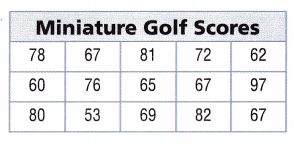Step 1
Write a title for the stem-and-leaf plot
Step 2
Use the tens digits for the stems.
Write the tens digits in order from least to greatest.5 – 3,
6 – 0,2,5,7,7,7,9
7 – 2,6,8
8 – 0,1,2
9 – 7.

Explanation:
The tens digits in order from least to greatest is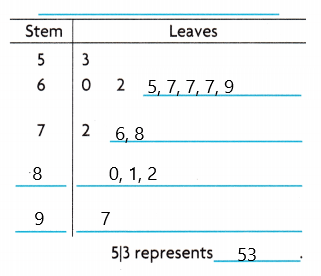Step 3 Use the ones digits for the leaves. Write the ones digits for each stem in order from least to greatest.
Step 4 Make a key.

• Describe how the number 80 is shown on the stem-and-leaf plot
8 is steam and 0 is a leaf.

Explanation:
The tens digit is 8 is a stem and the one’s digit is 0 is a leaf.

• Explain how the number that occurs most often in Jeremy’s record is shown on the stem-and-leaf plot. What is the number?
The number is 67.

Explanation:
There are 3 leaves of 7 for 6 stems 6. So the number is 67.

Try This! Make a stem-and-leaf plot with hundreds data.

A group of students do push-ups every morning before school. The number of push-ups they did for the past 12 mornings are 123, 141, 126, 120, 135, 136, 147, 129, 120, 130, 148, and 147. Make a stem-and-leaf plot of the data.• Write a title.
• Use the hundreds and tens digits to write the stems in order from least to greatest.
• Use the ones digits to write the leaves for each stem in order from least to greatest.

The title is Number of Push-Ups.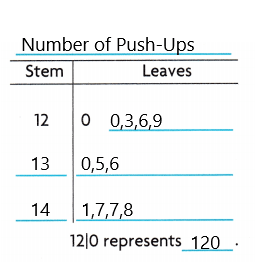• Explain how making a stem-and-leaf plot with hundreds data is similar to making a stem-and-leaf plot with tens data. Explain how it is different.
The similar is we will write the stems in order from least to greatest and we will use the ones digit for leaves of each stem.
The difference is we will use the hundreds and tens digits for the stems.

Share and Show

Ben keeps a record of the heights of his favorite basketball players. Use the data for 1-2.

Question 1.
What digits would you use for the stems in a stem-and-leaf plot of the data?

6,7,8.

Explanation:
The digits would we use for the stems in a stem-and-leaf plot of the data is 6,7,8.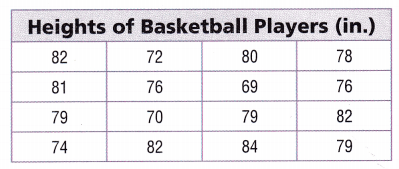How many leaves would a stem-and-leaf plot of the data have? ___
16.

Explanation:
The number of leaves would a stem-and-leaf plot of the data have is 16.

Question 2.
Make a stem-and-leaf plot of the data.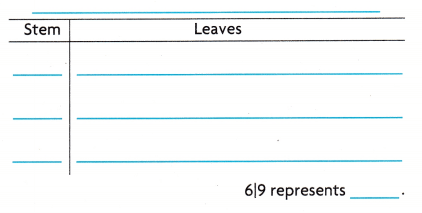The stem-and-leaf plot of the data isMath Talk
Mathematical Processes

Explain how to show a number that is a multiple of 10 on a stem-and-leaf plot.

Problem Solving

Question 3.
H.O.T. Representations Marco made a stem-and-leaf plot titled Game Scores. Each stem has at least 1 leaf. Ten numbers on the stem-and-leaf plot are less than 18 and 8 numbers are 18 or more. The number 10 occurs most often. Draw Marco’s stem-and-leaf plot.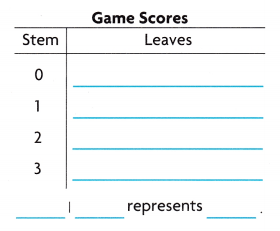Macro’s stem-and-leaf plot isQuestion 4.
Communicate How is a number less than 10 shown on a stem-and-leaf plot?
Here, the stem is 0 and the leaf is the number.

Problem Solving

Students were asked how many minutes it took them to get to school. Their responses in minutes were 25, 15, 29, 12, 30, 7, 26, 15, 23, 15, 21, 5, 27, 34, and 32. Use the data for 5-6.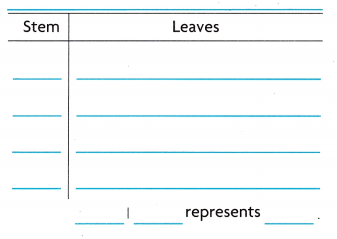Question 5.
Representations Make a stem-and-leaf plot of the data.
The stem-and-leaf plot of the data isQuestion 6.
Multi-Step If 5 and 7 minutes are changed to 45 and 47 minutes, how will the stem-and-leaf plot change?
There will be no stem or leaves for 0. There will be a stem for 4 with leaves for 5 and 7.

A salesperson earns a different amount of money each day. The amounts she earns in dollars are 325, 326, 311, 329, 325, 310, 301, 319, 329, 311, 304, 322, 311, 301, and 328. Use the data for 7-8.Question 7.
Explain how to determine what digits to use for stems in a stem-and-leaf plot of the data.
It is the place values of the numbers. The numbers are all hundreds, so we will use hundreds and tens digit for stems.

Question 8.
H.O.T. Multi-step Make a stem-and-leaf plot of the data.The stem-and-leaf plot of the data isQuestion 9.
Mark visits several bakeries. At each bakery, he asks how many cranberries they use to make a dozen cranberry muffins. Mark wants to use a stem-and-leaf plot to show the data. What are the leaves for the stem of 7?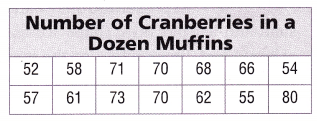(A) 0, 0, 1, 3, 5
(B) 0, 1, 3, 5
(C) 0, 1, 3
(D) 0, 0, 1, 3
D.

Explanation:
The leaves of the stem of 7 are 0, 0, 1, 3.

Use the table for 10 and 11.

Question 10.
Pam is making a stem-and-leaf plot to show the peach festival data. What is the fewest number of stems that she needs?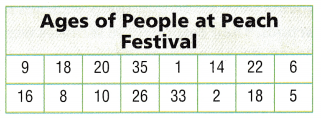(A) 3
(B) 2
(C) 4
(D) 5
4.

Explanation:
The fewest number of stems that she needs is 4 which are 0, 1, 2, 3.

Question 11.
Multi-Step How many more leaves will be on the stem of 1 than the stem of 3?
(A) 2
(B) 1
(C) 4
(D) 3
D.

Explanation:
The number of more leaves will be on the stem of 1 than the stem of 3 are 3.

Texas Test Prep

Question 12.
Mavis has 19 crossword puzzle books. The number of puzzles in each book is 42, 17, 20, 25, 43, 36, 42, 30, 29, 19, 22, 28, 47, 35, 42, 18, 20, 45, and 31. She makes a stem-and-leaf plot of the data. Which stem will have 4 leaves?
(A) stem 3
(B) stem 1
(C) stem 2
(D) stem 4
A.

Explanation:
The stem that has 4 leaves are stem 3 which are 0, 1, 5, 6.

### Texas Go Math Grade 5 Lesson 16.3 Homework and Practice Answer Key

The number of points scored by the members of a basketball team are recorded. Use the data for 1-2.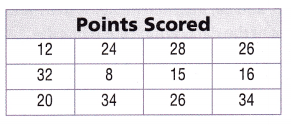Question 1.
What digits would you use for the stems in a stem-and-leaf plot of the data? ___
How many leaves would a stem-and-leaf plot of the data have? ____

Explanation:
The digits that could we use for the stems in a stem-and-leaf plot of the data are 0,1, 2, 3.

Question 2.
Make a stem-and-leaf plot of the data.Question 3.
Explain the difference between stems and leaves on a stem-and-leaf plot.

Problem Solving

Bird watchers were asked how many different species of birds they identified on a recent trip. Their responses were 15, 20, 30, 32, 21, 33, 18, 19, 29, 27, 29, 25, 21, and 26. Use the data for 4-5.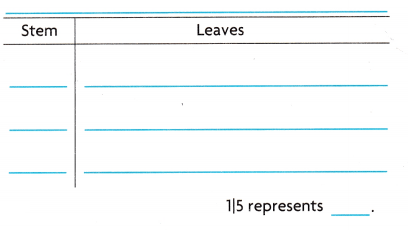Question 4.
Make a stem-and-leaf plot of the data.

Question 5.
If two more bird watchers identify 9 species and 41 species, how will the stem-and-leaf plot change?

Texas Test Prep

Lesson Check

Question 6.
Maxwell has 14 books in the same mystery series. The number of pages in each book are 112, 108, 95, 88, 96, 105, 115, 114, 85, 87, 90, 96, 116, and 98. Maxwell makes a stem-and-leaf plot of the data. Which stem has the most leaves?
(A) 9
(B) 11
(C) 10
(D) 8

Question 7.
Zara counts the number of raisins in several boxes of raisins. She wants to make a stem-and-leaf plot to show the data.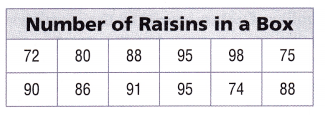What are the leaves for the stem 9?
(A) 0, 1, 5, 8
(B) 0, 1, 5, 5, 8
(C) 1, 5, 8
(D) 1, 5, 5, 8

Lincoln Heights Elementary held a student council election. The data show the number of votes each candidate received. Wesley makes a stem-and-leaf plot of the data. Use the table for 8-11.Question 8.
What is the number of stems that Wesley needs?
(A) 15
(B) 5
(C) 4
(D) 3

Question 9.
Which statement about the stem-and-leaf plot is true?
(A) There will be more stems than leaves.
(B) The stem 6 will have only 1 leaf.
(C) There will be 15 leaves.
(D) The number that occurs most often is 4.